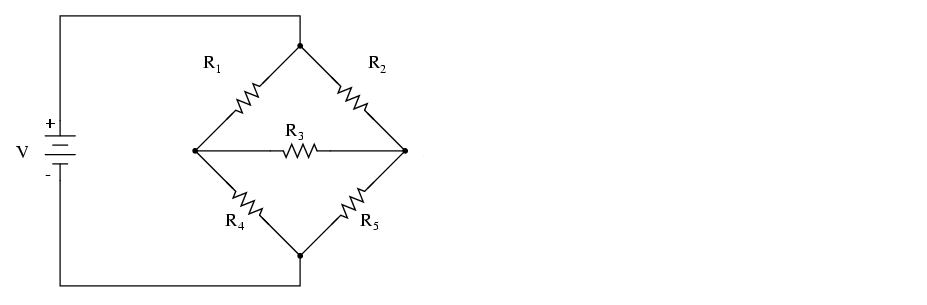# Problem: In the following figure, the configuration of resistors is known as a Wheatstone Bridge, which is used to measure the resistance of an unknown resistor (let's say R1 in our case). This is accomplished by varying the resistance of one of the outside resistors (let's say R5 in our case) until the resistance through the center of the bridge is zero. For our bridge, the battery produces 20 V and the fixed resistances are R2 = 10 Ω, R3 = 20 Ω, and R4 = 30 Ω. If the current through the center of the bridges is zero, and the current produced by the battery is 1 A, when R5 reaches a value of 15 Ω, what is the unknown resistance?

###### FREE Expert Solution
85% (219 ratings)
###### Problem Details

In the following figure, the configuration of resistors is known as a Wheatstone Bridge, which is used to measure the resistance of an unknown resistor (let's say R1 in our case). This is accomplished by varying the resistance of one of the outside resistors (let's say R5 in our case) until the resistance through the center of the bridge is zero. For our bridge, the battery produces 20 V and the fixed resistances are R2 = 10 Ω, R3 = 20 Ω, and R4 = 30 Ω. If the current through the center of the bridges is zero, and the current produced by the battery is 1 A, when R5 reaches a value of 15 Ω, what is the unknown resistance?Frequently Asked Questions

What scientific concept do you need to know in order to solve this problem?

Our tutors have indicated that to solve this problem you will need to apply the Kirchhoff's Loop Rule concept. You can view video lessons to learn Kirchhoff's Loop Rule. Or if you need more Kirchhoff's Loop Rule practice, you can also practice Kirchhoff's Loop Rule practice problems.

How long does this problem take to solve?

Our expert Physics tutor, Juan took 5 minutes and 10 seconds to solve this problem. You can follow their steps in the video explanation above.

What professor is this problem relevant for?

Based on our data, we think this problem is relevant for Professor Khondaker's class at UCF.